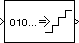Uniform Decoder

Decode integer input into floating-point output

Library

Quantizers

dspquant2

•Description

The Uniform Decoder block performs the inverse operation of the Uniform Encoder block, and reconstructs quantized floating-point values from encoded integer input. The block adheres to the definition for uniform decoding specified in ITU-T Recommendation G.701.

Inputs can be real or complex values of the following six integer data types: uint8, uint16, uint32, int8, int16, or int32.

The block first casts the integer input values to floating-point values, and then uniquely maps (decodes) them to one of 2B uniformly spaced floating-point values in the range [-V, (1-21-B)V], where you specify B in the Bits parameter (as an integer between 2 and 32) and V is a floating-point value specified by the Peak parameter. The smallest input value representable by B bits (0 for an unsigned input data type; -2B-1 for a signed input data type) is mapped to the value -V. The largest input value representable by B bits (2B-1 for an unsigned input data type; 2B-1-1 for a signed input data type) is mapped to the value (1-21-B)V. Intermediate input values are linearly mapped to the intermediate values in the range [-V, (1-21-B)V].

To correctly decode values encoded by the Uniform Encoder block, the Bits and Peak parameters of the Uniform Decoder block should be set to the same values as the Bits and Peak parameters of the Uniform Encoder block. The Overflow mode parameter specifies the Uniform Decoder block's behavior when the integer input is outside the range representable by B bits. When you select Saturate, unsigned input values greater than 2B-1 saturate at 2B-1; signed input values greater than 2B-1-1 or less than -2B-1 saturate at those limits. The real and imaginary components of complex inputs saturate independently.

When you select Wrap, unsigned input values, u, greater than 2B-1 are wrapped back into the range [0, 2B-1] using mod-2B arithmetic.

u = mod(u,2^B)

Signed input values, u, greater than 2B-1-1 or less than -2B-1 are wrapped back into that range using mod-2B arithmetic.

u = (mod(u+2^B/2,2^B)-(2^B/2))

The real and imaginary components of complex inputs wrap independently.

The Output type parameter specifies whether the decoded floating-point output is single or double precision. Either level of output precision can be used with any of the six integer input data types.

Examples

See example model ex_uniform_decoder.

In this example, the input to the block is the uint8 output of a Uniform Encoder block. This block has comparable settings: Peak = 2, Bits = 3, and Output type = Unsigned. (Comparable settings ensure that inputs to the Uniform Decoder block do not saturate or wrap. See the example on the Uniform Encoder block reference page for more about these settings.)

The real and complex components of each input are independently mapped to one of 23 distinct levels in the range [-2.0,1.5].

0  is mapped to  -2.0
1  is mapped to  -1.5
2  is mapped to  -1.0
3  is mapped to  -0.5
4  is mapped to   0.0
5  is mapped to   0.5
6  is mapped to   1.0
7  is mapped to   1.5

Parameters

Peak

Specify the largest amplitude represented in the encoded input. To correctly decode values encoded with the Uniform Encoder block, set the Peak parameters in both blocks to the same value.

Bits

Specify the number of input bits, B, used to encode the data. (This can be less than the total number of bits supplied by the input data type.) To correctly decode values encoded with the Uniform Encoder block, set the Bits parameters in both blocks to the same value.

Overflow mode

Specify the block's behavior when the integer input is outside the range representable by B bits. Out-of-range inputs can either saturate at the extreme value, or wrap back into range.

Output type

Specify the precision of the floating-point output, single or double.

References

General Aspects of Digital Transmission Systems: Vocabulary of Digital Transmission and Multiplexing, and Pulse Code Modulation (PCM) Terms, International Telecommunication Union, ITU-T Recommendation G.701, March, 1993

Supported Data Types

PortSupported Data Types

Input

• 8-, 16-, and 32-bit integers

Output

• Double-precision floating point

• Single-precision floating point

 Data Type Conversion (Simulink) Simulink Quantizer (Simulink) Simulink Scalar Quantizer Decoder DSP System Toolbox Uniform Encoder DSP System Toolbox udecode Signal Processing Toolbox uencode Signal Processing Toolbox

Extended Capabilities

C/C++ Code GenerationGenerate C and C++ code using Simulink® Coder™.

Introduced before R2006a

DSP System Toolbox DocumentationGet trial now